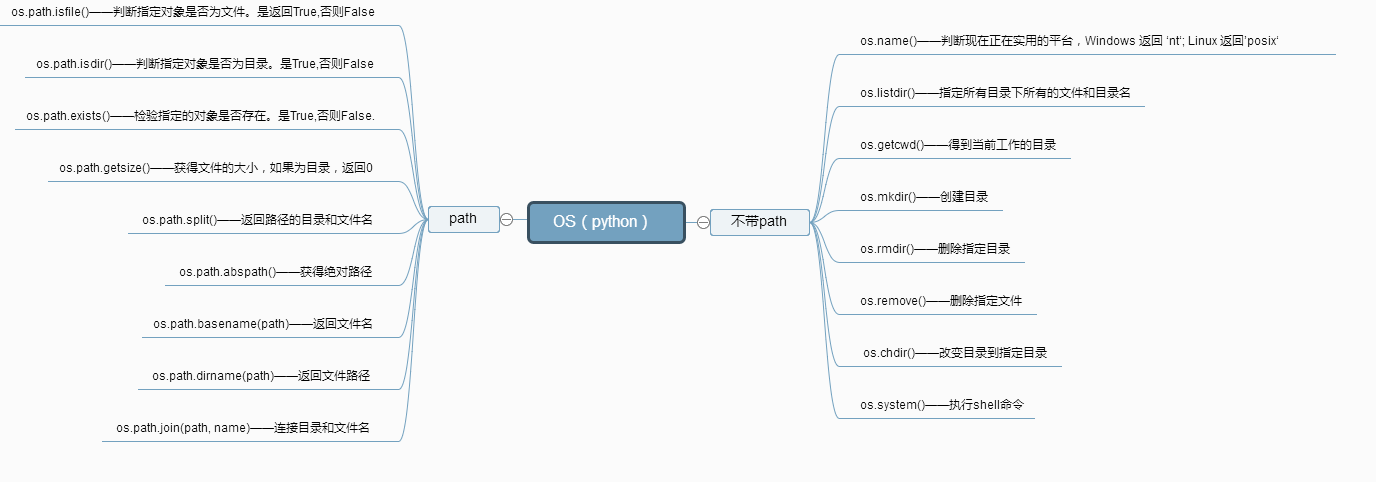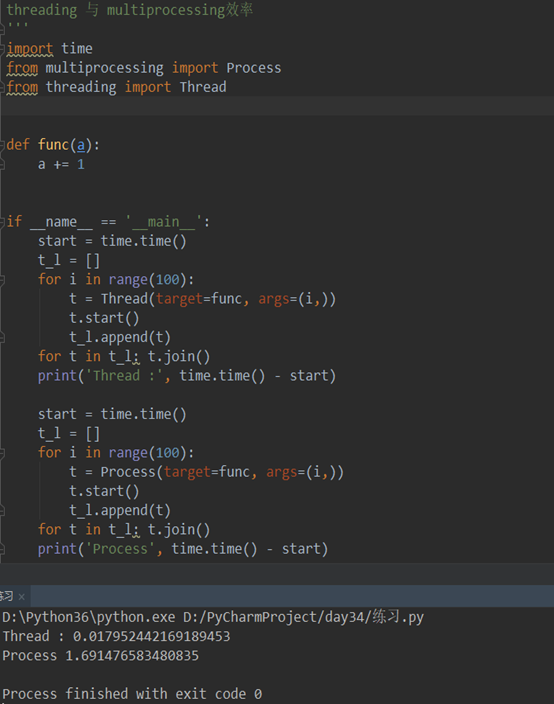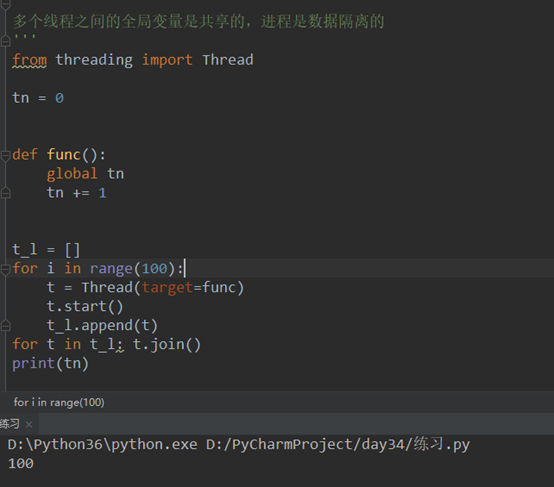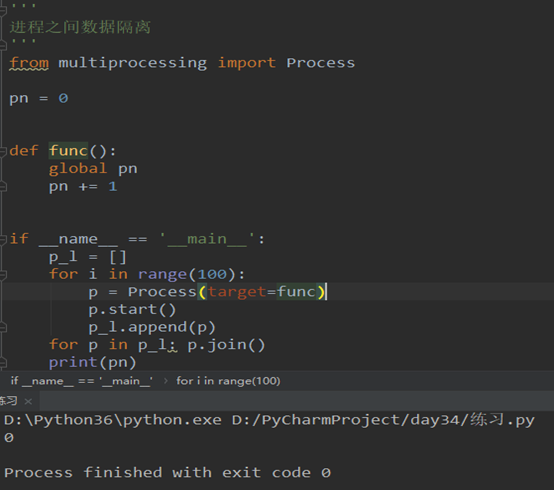2019/03/19 20:07

# 模块

## 模块对我来说是什么

模块对我来说，感觉就像亲属或者朋友已经走过的路，他们已经趟过的浑水、掉过的坑、践行过的路线，全部提供给你，在你需要的时候请求帮助，借鉴他们的解决方法一样。都是为了方便走好人生路，用最短的路程走到成功的终点。

内置模块就像亲属，生来即有；自定义模块就像自己走过一次的路，吃一堑长一智做好了攻略，下次按攻略来就可以；第三方模块就像之后接触到的朋友，他们成功的经验借鉴给你，自己选择，规避坑陷。

## 模块调用的方法

import 模块名

from 模块名 import 方法名

## emmm……python官方的开源模块库下载地址（防遗忘）

https://pypi.python.org/pypi

## 内置模块

### time/datetime模块（还有一个calendar模块，只了解过）

处理与时间有关的，感觉目前时间模块我多用于测试（例如：time.sleep( )）和日志中。

在写方法前先解释下时间戳、结构化时间、字符串时间这些概念，当然也防止自己以后再看到时间戳的时候怀疑自己这是个啥玩意儿。

时间戳：1970纪元后经过的浮点秒数。

结构化时间：输出结果是这种的，以时间是由什么结构组成的输出，总之方便调用或修改。（tm_year,tm_mon,tm_mday,tm_hour,tm_min,tm_sec,tm_wday,tm_yday,tm_isdst）

字符串时间：就是正常显示的时间

time.time()：获取当前时间的时间戳

这里例一个字符串时间t：

``````t='2019-01-01 10:00:00'

f=time.strptime(t,'%Y-%m-%d %X')  这一步是将字符串时间t转为结构化

f=time.mktime(f)   这一步是将结构化时间转为时间戳

f=time.localtime(f)        这一步是将时间戳转成结构化时间

f=time.strftime('%Y-%m-%d %X',f) 将结构化时间转为字符串时间``````

time是datetime的底层模块，貌似我不怎么用到，这里就不详写了。

``````from datetime import datetime,timedelta

# import datetime

# print(datetime.now())

# # 时间对象

# f=datetime.now()

# a=datetime.timestamp(datetime.now())  #将时间对象转换成时间戳

# print(datetime.fromtimestamp(a))    #将时间戳转成时间对象

print(datetime.now()-timedelta(days=2))``````

### random、hmac模块（随机模块，做验证客户端合法性的hmac模块）

random模块特别重要，实现随机数功能，或随机抽取这种的都需要用到它。

``````import random     内置的

print(random.random())      0-1之间随机小数

print(random.random(1，10))     起始位置，终止位置 两头都包含

print(random.random(1，11，2)) 起始位置，终止位置（不包含），步长

print(random.choice([1,2,2]))   从可迭代、有序对象中随机选

print(random.choices([1,2,3]，k=2))   选择两个，但有重复

print(random.sample([1,2,3]，k=2))    选择两个，没有重复

li=[1,2,3,4,6]

random.shuffle(li)  洗牌 打乱顺序

print(li)``````

hmac模块（做验证客户端合法性的，虽然我觉得它有可能不止用在验证合法性。）

os.urandom(32)随机的32位字节，每执行一次变一次

``````import os

import hmac

hmac=hmac.new(b’zhao’,os.urandom(32))

hmac.digest()：以固定字节内容和随机内容组合加密，然后与客户端进行相同方法加密比较，如果相同则合法。``````

### os模块

``````得到当前工作目录，即当前Python脚本工作的目录路径: os.getcwd()### sys模块

``````sys.argv           命令行参数List，第一个元素是程序本身路径.之后的元素会传入程序本身。第二个元素在sys.argv列表中索引为【1】

sys.exit(n)        退出程序，正常退出时exit(0)

sys.version        获取Python解释程序的版本信息

sys.maxint         最大的Int值

sys.path           返回模块的搜索路径，初始化时使用PYTHONPATH环境变量的值

sys.platform       返回操作系统平台名称

sys.getrecursionlimit() #获取最大递归层数

sys.setrecursionlimit(1200) #设置最大递归层数

sys.getdefaultencoding()  #获取解释器默认编码

sys.getfilesystemencoding  #获取内存数据存到文件里的默认编码``````

### 序列化模块（json/pickle/shelve模块）

import json/pickle/shelve

json模块：

``````json.load()      将文件中的字符串转换成字典

json.dump() 将字典转换成字符串写入到文件中

json.dumps() 将字典转换成字符串

pickle模块：

``````pickle.load()   将文件中的字节转换成字典

pickle.dump() 将字典转换成字节写入到文件中

pickle.dumps() 将字典转换成字节

json和pickle的优缺点：

json

优点：跨语言、体积小

缺点：只能支持Int\str\list\tuple\dict

pickle:

优点：专为python设计，支持python所有的数据类型

缺点：只能在python中使用，存储数据占空间大

shelve模块（不怎么了解，copy的）：

shelve模块是一个简单的k,v将内存数据通过文件持久化的模块，可以持久化任何pickle可支持的python数据格式

序列化：

``````import shelve

f = shelve.open('shelve_test')  # 打开一个文件

names = ["alex", "rain", "test"]

info = {'name':'alex','age':22}

f["names"] = names  # 持久化列表

f['info_dic'] = info

f.close()``````

``````import shelve

d = shelve.open('shelve_test')  # 打开一个文件

print(d['names'])

print(d['info_dic'])

#del d['test'] #还可以删除``````

### hashlib模块（加密）

hashlib包括：MD5，sha1，sha256，sha512

1. 先导入模块
2. 创建一个加密方式
3. 将要加密的内容编码成字节后加密
4. 生成密文
``````import hashlib

md5 = hashlib.md5(b‘alex’)

md5.update(‘alex3714’.encode(‘utf-8’))

print(md5.hexdigest())``````

### logging模块（日志模块）

日志模块可以记录正常的访问操作日志，而且可以记录错误、警告等信息，可以更直观的告诉开发应该注意哪里的问题。

python的logging模块提供了标准的日志接口，你可以通过它存储各种格式的日志，logging的日志可以分为 debug(), info(), warning(), error() and critical()5个级别，下面我们看一下怎么用。

一个卡务系统 : 记录时间 地点 金额

谁在什么时候 删掉了某一个用户

某时某刻登录了系统

检测代码是不是像我们想像的这样执行的

f.write,print

*** logging模块不能自动生成你需要的日志

logging模块的使用

简单配置法      ***  编码问题

logger对象法   *****

``````import logging

logging.basicConfig(level=logging.DEBUG,

format='%(asctime)s %(filename)s[line:%(lineno)d] %(levelname)s %(message)s',

datefmt='%a, %d %b %Y %H:%M:%S')

logging.debug('debug message')      # 调试

logging.info('info message')        # 普通信息

logging.warning('warning message')  # 警告

logging.error('error message')      # 错误信息

logging.critical('critical message')# 严重错误``````

1.中文显示乱码

2.不能同时输出到文件和屏幕

logger对象的方式来使用logging模块

logger 绑定 文件操作符

logger 绑定 屏幕操作符

import logging

logger = logging.getLogger()

logger.setLevel(logging.DEBUG)

fh = logging.FileHandler('log',encoding='utf-8')

sh = logging.StreamHandler()

``````fmt = logging.Formatter('%(asctime)s - %(name)s - %(levelname)s - %(message)s')

logger 绑定 文件操作符

logger 绑定 屏幕操作符

fh.setFormatter(fmt)

sh.setFormatter(fmt)

logger.debug('logger debug message')

logger.info('logger info message')

logger.warning('logger warning message')

logger.error('logger error message')

logger.critical('logger critical message')``````

### re模块（正则）

'.' ：默认匹配除\n之外的任意一个字符

'^'：匹配字符开头

'\$' ：匹配字符结尾

'*' ：匹配*号前的字符0次或多次

'+' ：匹配前一个字符1次或多次

'?' 匹配前一个字符1次或0次

'{m}' ：匹配前一个字符m次

'{n,m}' ：匹配前一个字符n到m次，re.findall("ab{1,3}","abb abc abbcbbb") 结果'abb', 'ab', 'abb']

'|' ：匹配|左或|右的字符，re.search("abc|ABC","ABCBabcCD").group() 结果'ABC'

'(...)' ：分组匹配，re.search("(abc){2}a(123|45)", "abcabca456c").group() 结果为'abcabca45'

'\A' 只从字符开头匹配

'\Z' ：匹配字符结尾，同\$

'\d' ：匹配数字0-9

'\D' ：匹配非数字

'\w' ：匹配[A-Za-z0-9]

'\W' ：匹配非[A-Za-z0-9]

's' ：匹配空白字符

'(?P<name>...)' ：分组匹配

re的匹配语法：

``````ret=re.findall('正则表达式','内容')

print(ret)``````

``````ret=re.search('正则表达式','内容')

if ret:

print(ret.group())``````

``````ret=re.match('正则表达式','内容')

print(ret.group())``````

``````ret=re.sub(‘正则表达式’,’新值’,’完整字符串’)

ret=re.sub(‘正则表达式’,’新值’,’完整字符串’,’n’)``````

``````ret=re.subn(‘正则表达式’,’新值’,’完整字符串’)

print(ret)``````

``ret=re.split(‘\d+’,’内容’)``

### abc模块（抽象类）

``````#一切皆文件
import abc #利用abc模块实现抽象类

class All_file(metaclass=abc.ABCMeta):
all_type='file'
@abc.abstractmethod #定义抽象方法，无需实现功能
'子类必须定义读功能'
pass

@abc.abstractmethod #定义抽象方法，无需实现功能
def write(self):
'子类必须定义写功能'
pass

# class Txt(All_file):
#     pass
#
# t1=Txt() #报错,子类没有定义抽象方法

print('文本数据的读取方法')

def write(self):
print('文本数据的读取方法')

print('硬盘数据的读取方法')

def write(self):
print('硬盘数据的读取方法')

print('进程数据的读取方法')

def write(self):
print('进程数据的读取方法')

wenbenwenjian=Txt()

yingpanwenjian=Sata()

jinchengwenjian=Process()

#这样大家都是被归一化了,也就是一切皆文件的思想
yingpanwenjian.write()

print(wenbenwenjian.all_type)
print(yingpanwenjian.all_type)
print(jinchengwenjian.all_type)``````

### multiprocessing模块（多进程模块）

``````import time

from multiprocessing import Process

def func(a,b,c):

time.sleep(1)

print(a,b,c)

if __name__ == '__main__':

Process(target=func,args=(1,2,3)).start()        #这里的target是目标的意思，固定搭配

Process(target=func,args=(2,3,4)).start()        #所有的Process（）都是子进程，子进程都放在if下。

Process(target=func,args=(3,4,5)).start()``````

需要注意的是：下面标红区域必须是元组，当只传一个值时，必须也为元组形式。

``````import time

from multiprocessing import Process

def func(a):

time.sleep(1)

print(a)

if __name__ == '__main__':

Process(target=func,args=(1,)).start()

Process(target=func,args=(2,)).start()``````

multiprocessing中的方法：

Lock

Queue    队列

Manager       共享资源

Manager类的作用共享资源，manger的的优点是可以在poor进程池中使用，缺点是windows下环境下性能比较差，因为windows平台需要把Manager.list放在if name='main'下，而在实例化子进程时，必须把Manager对象传递给子进程，否则lists无法被共享，而这个过程会消耗巨大资源，因此性能很差。

没有池的功能

实现了池的功能

concurrent.futures

实现了线程池\进程池

``````import os

import time

def func(i):

time.sleep(1)

print('in func', i,os.getpid())

print('in main', os.getpid())

for i in range(20):

``````import time

from multiprocessing import Process

def func(a):

a += 1

if __name__ == '__main__':

start = time.time()

t_l = []

for i in range(100):

t.start()

t_l.append(t)

for t in t_l: t.join()

start = time.time()

t_l = []

for i in range(100):

t = Process(target=func, args=(i,))      #多进程

t.start()

t_l.append(t)

for t in t_l: t.join()

print('Process', time.time() - start)````````````from threading import Thread

tn = 0

def func():

global tn

tn += 1

t_l = []

for i in range(100):

t.start()

t_l.append(t)

for t in t_l: t.join()

print(tn)````````````from multiprocessing import Process

pn = 0

def func():

global pn

pn += 1

if __name__ == '__main__':

p_l = []

for i in range(100):

p = Process(target=func)

p.start()

p_l.append(p)

for p in p_l: p.join()

print(pn)````````````import os

def func():

print(t.name, t.ident, os.getpid())

tobj.start()

print('tobj :', tobj)

print(t2.name, t2.ident, os.getpid())``````

没有terminate 不能强制结束

所有的子线程都会在执行完所有的任务之后自动结束

0
0 收藏

### 作者的其它热门文章0 评论
0 收藏
0